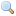# Module Arith_status

`module Arith_status: `sig` .. `end``
Flags that control rational arithmetic.

`val arith_status : `unit -> unit``
Print the current status of the arithmetic flags.
`val get_error_when_null_denominator : `unit -> bool``
`val set_error_when_null_denominator : `bool -> unit``
Get or set the flag `null_denominator`. When on, attempting to create a rational with a null denominator raises an exception. When off, rationals with null denominators are accepted. Initially: on.
`val get_normalize_ratio : `unit -> bool``
`val set_normalize_ratio : `bool -> unit``
Get or set the flag `normalize_ratio`. When on, rational numbers are normalized after each operation. When off, rational numbers are not normalized until printed. Initially: off.
`val get_normalize_ratio_when_printing : `unit -> bool``
`val set_normalize_ratio_when_printing : `bool -> unit``
Get or set the flag `normalize_ratio_when_printing`. When on, rational numbers are normalized before being printed. When off, rational numbers are printed as is, without normalization. Initially: on.
`val get_approx_printing : `unit -> bool``
`val set_approx_printing : `bool -> unit``
Get or set the flag `approx_printing`. When on, rational numbers are printed as a decimal approximation. When off, rational numbers are printed as a fraction. Initially: off.
`val get_floating_precision : `unit -> int``
`val set_floating_precision : `int -> unit``
Get or set the parameter `floating_precision`. This parameter is the number of digits displayed when `approx_printing` is on. Initially: 12.